# How to count cells by criteria

Excel
Use COUNTIF if you need to sum values for a particular person or another criterion.

To count cells by criteria, do the following:

1.   Select the cell where you want Excel to return the cell number by criteria.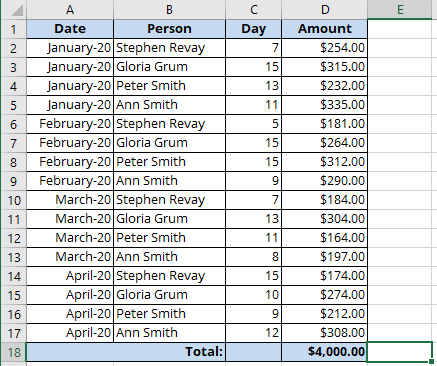2.   Do one of the following:

• On the Formula tab, in the Function Library group, select the More Functions button and then select Statistical: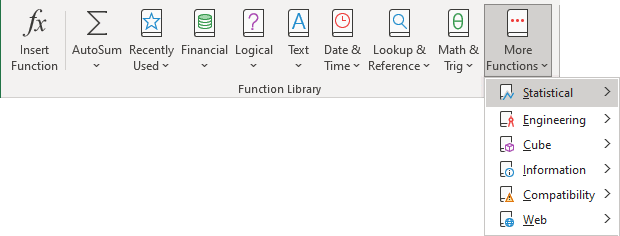Choose COUNTIF in the list.

• Click the Insert Function button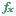on the left of the Formula bar: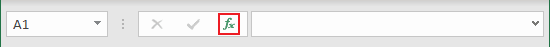In the Insert Function dialog box:

• select Statistical in the Or select a category drop-down list,
• select COUNTIF in the Select a function list: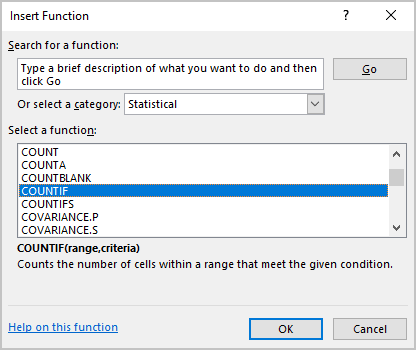3.   In the Function Arguments dialog box: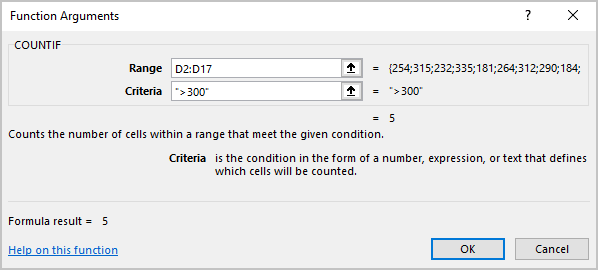• The Range field determines the range of cells Excel will look to perform the count in. In this example, the cell range is D2:D17.
• The Criteria is a conditional statement similar to the conditional statement in the IF statement.

4.   Press OK: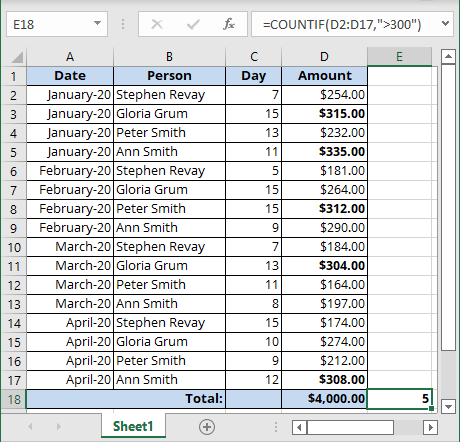Notes:

• You can enter this formula using the keyboard, for this example:

= COUNTIF (D2:D17, ">300")

• If you want to use COUNTIF on a selection of cells (not necessarily one solid range), summing multiple COUNTIFs:

= SUM (COUNTIF (D3:D7, ">200"), COUNTIF (D14:D17, ">200"))

• You can use the wildcard characters: the question mark (?) and the asterisk (*) in the criteria. A question mark matches any single character; an asterisk matches any sequence of characters. If you want to find an actual question mark or asterisk, type a tilde (~) before the character.
• Microsoft Excel provides additional functions that can be used to analyze your data based on a condition.
• To calculate a sum based on a string of text or a number within a range, use the SUMIF worksheet function (see How to sum cells by criteria for more details).
• To have a formula return one of two values based on a condition, such as a sales bonus based on a specified sales amount, use the IF worksheet function.
• To count cells that are empty or not empty, use the COUNTA and COUNTBLANK functions.

See also this tip in French: Comment compter les cellules par critères.